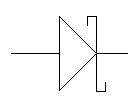# Electronics and Communication Engineering - Power Electronics

### Exercise :: Power Electronics - Section 3

11.

A single phase half wave converter is used to charge a battery of 50 V. The thyristor is continuously fired by dc signal. Input voltage is v = Vm sin ωt. The thyristor will conduct

 A. from 0 to 180° B. when v > 50 V C. from 0 to 90° D. When v < 50 V

Explanation:

Thyristor can conduct only when it is forward biased. This occurs only when v > 50 V.

12.

The symbol in figure is forA. DIAC B. P-N junction diode C. Schottky diode D. None of the above

Explanation:

No answer description available for this question. Let us discuss.

13.

In a 3 phase semiconverter, the firing frequency is

 A. equal to line frequency B. 3 times the line frequency C. 6 times the line frequency D. 12 times the line frequency

Explanation:

Since the circuit has 3 thyristors, firing frequency is three times the line frequency.

14.

Present day HVDC converters are all

 A. 3 pulse converters B. 6 pulse converters C. 12 pulse converters D. either 6 pulse or 12 converters

Explanation:

To reduce ripple 12 pulse converters are used.

15.

A single phase full converter gives maximum and minimum output voltage at firing angles of

 A. 0 and 180° respectively B. 180° and 0° respectively C. 0 and 90° respectively D. 90° and 0° respectively